## Open Conductor Faults:

An open conductor faults is in series with the line. Line currents and series voltages between broken ends of the conductors are required to be determined.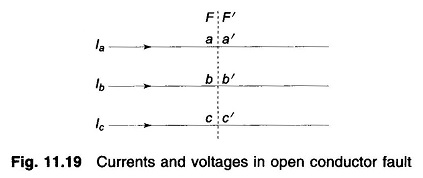Figure 11.19 shows currents and voltages in an open conductor faults. The ends of the system on the sides of the fault are identified as F, F′, while the conductor ends are identified as aa′, bb′ and cc′. The set of series currents and voltages at the fault are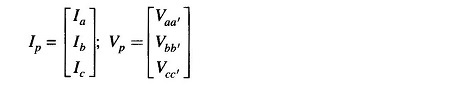The symmetrical components of currents and voltages are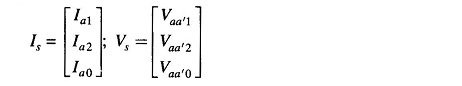The sequence networks can be drawn for the power system as seen from FF′ and are schematically shown in Fig. 11.20. These are to be suitably connected depending on the type of fault (one or two conductors open).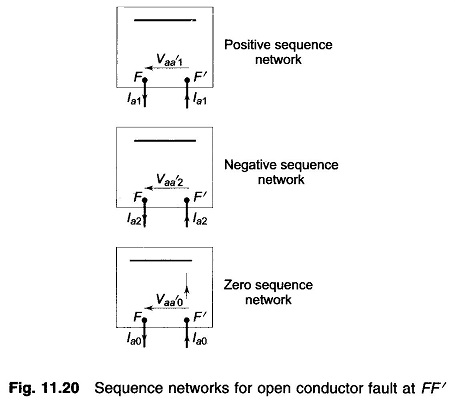### Two Conductors Open: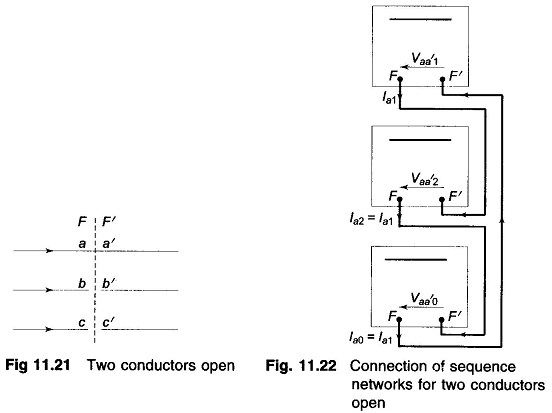Figure 11.21 represents the fault at FF′ with conductors b and c open. The currents and voltages due to this fault are expressed as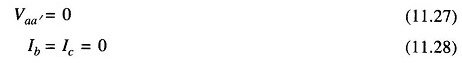In terms of symmetrical components, we can write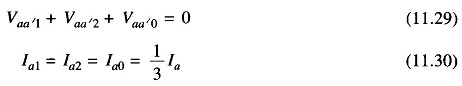Equations (11.29) and (11.30) suggest a series connection of sequence networks as shown in Fig. 11.22. Sequence currents and voltages can now be computed.

### One Conductor Open: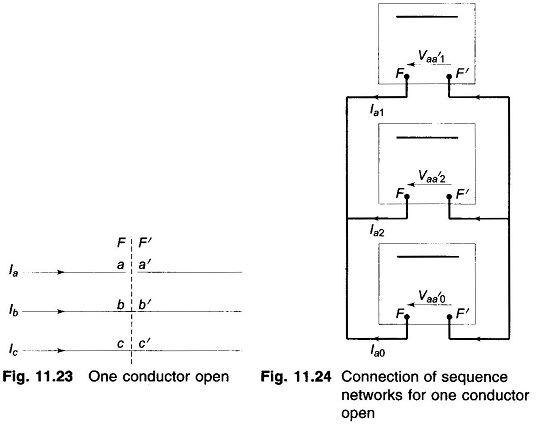For one conductor open as in Fig. 11.23, the circuit conditions require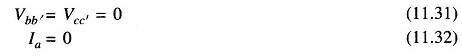In terms of symmetrical components these conditions can be expressed as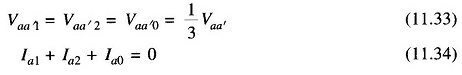Equations (11.33) and (11.34) suggest a parallel connection of sequence networks as shown in Fig. 11.24.

Scroll to Top